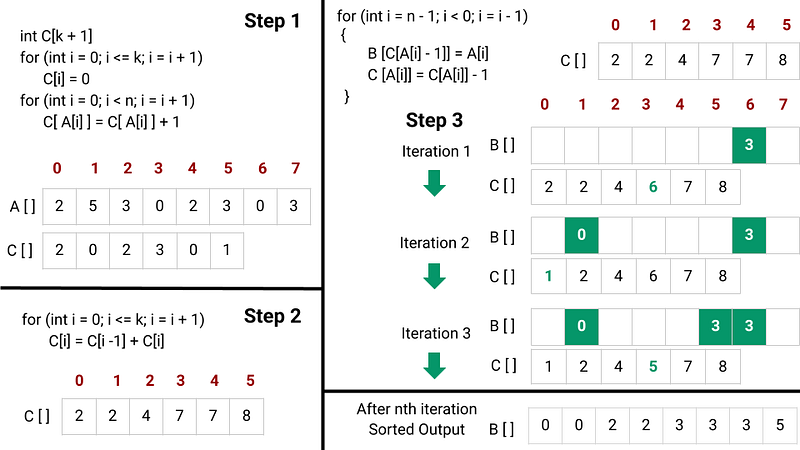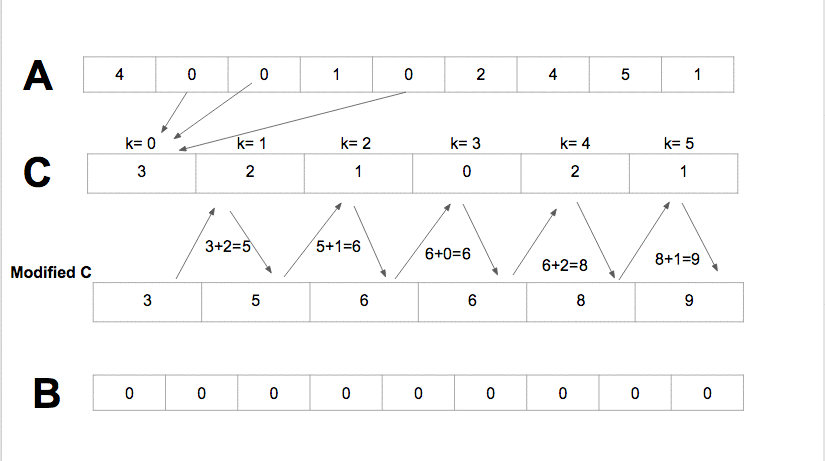# Counting Sort

As we have seen in this blog that the lower bound of comparison sorting is O(nlogn). Still, suppose we can gather information about the sorted order of the input by means other than comparing elements. In that case, we can improve the time complexity of the sorting algorithm from O(nlogn) to O(n). The counting sort algorithm, for example, assumes that each of the n input elements is an integer in the range 0 to k. So by using array indexing as a tool for determining relative order, counting sort can sort n numbers in O(k + n) time when k = O(n). In other words, counting sort is one of the popular linear time sorting algorithms that works in O(n) time complexity if input elements are an integer in the range 0 to k.

Counting Sort Idea

The basic idea of counting sort is to determine, for each input element x, the number of elements less than x. This information can be used to place element x directly into its position in the output array.

For example, if there are 17 elements less than x, then x belongs in output position 18. This scheme must be modified slightly to handle the situation in which several elements have the same value since we don’t want to put them all in the same position.

Counting Sort Steps

• We use two extra arrays: the array B[n] to holds the sorted output and the count array C[k + 1] to store the count of each unique input value available in the range 0 to k. We initialize all values of the count array C[] to zeros using a loop.

``````for (int i = 0; i <= k; i = i + 1)
C[i] = 0
``````
• Now we scan the input array A[] from start to end and store the count of each value in the count array C[]. Here we store the count of value A[i] at index A[i] in the C[]. In other words, we inspect each input element using a loop. If the value of an input element is i, we increment the value at C[i]. So by the end of this process, C[i] holds the number of input elements equal to i for each integer i = 1 to k.

``````for (int i = 0; i < n; i = i + 1)
C[A[i]] = C[A[i]] + 1
``````
• Now we determine for each i = 0 to k how many input elements are less than or equal to i by keeping a running sum of the array C. In other words, we modify the count array C[] such that each element at each index stores the sum of previous counts. The modified count array indicates the position of each element in the sorted output.

``````for (int i = 0; i <= k; i = i + 1)
C[i] = C[i - 1] + C[i]
``````
• Now using a loop, we place each element A[i] into its correct sorted position in the output array B[]. If all n elements are distinct, then for each A[i], the value C[A[i]] - 1 is the correct final index of A[i] in the output array since there are C[A[i]] elements less than or equal to A[i]. Because the elements might not be distinct, we decrement C[A[i]] each time we place a value A[i] into the B[] array. Decrementing C[A[i]] causes the next input element with a value equal to A[i], if one exists, to go to the position immediately before A[i] in the output array.

``````for (int i = n - 1; i < 0; i = i - 1)
{
B[C[A[i]] - 1] = A[i]
C[A[i]] = C[A[i]] - 1
}
``````
• By the end of the above process, our sorted output will be stored in the array B[].

Counting Sort Pseudocode

``````void countingSort(int A[], int B[], int k, int n)
{
int C[k + 1]
for (int i = 0; i <= k; i = i + 1)
C[i] = 0
for (int i = 0; i < n; i = i + 1)
C[A[i]] = C[A[i]] + 1

for (int i = 0; i <= k; i = i + 1)
C[i] = C[i - 1] + C[i]

for (int i = n - 1; i < 0; i = i - 1)
{
B[C[A[i]] - 1] = A[i]
C[A[i]] = C[A[i]] - 1
}
}
``````

Counting Sort Pseudocode VisualizationCounting Sort VisualizationGIF reference: brilliant.org

Time and space complexity analysis

There are four loops in the counting sort algorithm, where:

• The first loop takes O(k) time
• The second loop takes O(n) time
• The third loop takes O(k) time
• The fourth loop takes O(n) time

Therefore, the overall time of the counting sort = O(k) + O(n) + O(k) + O(n) = O(k + n), If k = O(n), then time complexity = O(n)

Space Complexity = Size of output[] array + size of count[] array = O(n) + O(k) = O(n + k), If k = O(n), then space complexity = O(n)

### Properties of counting sort

• The time and space complexity of the counting sort depends on the range of the input data k. It is efficient if the range of input value is not significantly greater than the number of elements to be sorted. If k = O(n^2), then the time and space complexity of the counting sort will be O(n^2)
• Counting sort beats the lower bound of O(nlogn) because it is not a comparison sort. No comparisons between input elements occur anywhere in the code. Instead, counting sort uses the actual values of the elements to index into an array. The O(nlogn) lower bound for sorting does not apply when we depart from the comparison sort model.
• An important property of counting sort is that it is stable: numbers with the same value appear in the output array in the same order as in the input array. It breaks ties between two numbers by the rule that whichever number appears first in the input array appears first in the output array.
• Normally, the property of stability is important only when additional data are carried around with the element being sorted. Counting sort’s stability is important for another reason: counting sort is often used as a subroutine in radix sort.
• Counting sort uses the idea similar to the direct addressing method of hashing to count the occurrence of the input elements in O(1).

### Critical ideas to think!

• How do we extend the above algorithm to work for negative inputs also?
• Instead of running the last loop from i = n-1 to 0, How do we correctly modify the counting sort code to run it from i = 0 to n-1? Is the modified algorithm stable?
• How do we modify the above algorithm if input data to sort in the range from [a, b]?
• Describe an algorithm that, given n integers in the range 0 to k, preprocesses its input and then answers any query about how many n integers fall into a given range [a...b] in O(1) time. Your algorithm should use O(n + k) preprocessing time.

### Suggested concepts and problems to explore

Reference: Algorithms by CLRS

Enjoy learning, Enjoy thinking!

Get well-designed application and interview centirc content on ds-algorithms, machine learning, system design and oops. Content will be delivered weekly.